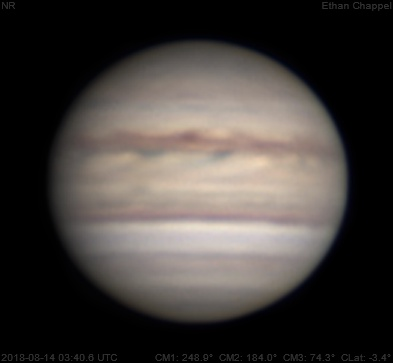# Jupiter 2018-08-14 03:40 UTC

CM1: 248.90°

CM2: 184.00°

CM3: 74.30°

CLat: -3.40°

Description

Soon after an exhausting two weeks of astrophotography in July, I went to the Green Bank Observatory in West Virginia to do some radio astronomy projects in ERIRA. I highly recommend looking at the program if you're a university student and have even a slight interest in astronomy and research. While I was there I calculated the mass of Jupiter using three of my images and landed less than one percent above the accepted value! Three days after returning from WV, I resumed imaging from home under average seeing and good transparency. I used my Tele Vue 2.5x Powermate for this and the next few nights.

Notes:

• The North Temperate Belt has almost disappeared.
• A brown barge on the North Equatorial Belt is near the central meridian.
• The Equatorial Zone is tinted orange.

Equipment

ZWO ASI224MC

Tele Vue 2.5x Powermate

Celestron EdgeHD 8

Logs
```FireCapture v2.6  Settings
------------------------------------
Observer=Ethan Chappel
Location=Cibolo, TX, USA
Scope=Celstron C14 EdgeHD
Camera=ZWO ASI224MC
Filter=RGB
Profile=Jupiter
Diameter=36.44"
Magnitude=-2.03
CMI=252.9° CMII=187.9° CMIII=78.3°  (during mid of capture)
FocalLength=3550mm (F/9)
Resolution=0.22"
Filename=2018-08-14-0346_4-EC-RGB-Jup.ser
Date=2018_08_14
Start=034528.942
Mid=034628.956
End=034728.970
Start(UT)=034528.942
Mid(UT)=034628.956
End(UT)=034728.970
Duration=120.028s
Date_format=yyyy_MM_dd
Time_format=HHmmss
LT=UT -6h
Frames captured=4000
File type=SER
Binning=no
Bit depth=8bit
Debayer=yes
DebayerAlgorithm=NearestNeighbor
BayerPattern=RG
ROI=364x328
ROI(Offset)=0x0
FPS (avg.)=33
Shutter=30.00ms
Gain=330 (55%)
HardwareBin=off
HighSpeed=off
AutoGain=off
WRed=55 (off)
AutoHisto=75 (off)
WBlue=95 (off)
FPS=100 (off)
Gamma=50 (off)
USBTraffic=100 (off)
AutoExposure=off
Brightness=1 (off)
SoftwareGain=10 (off)
Histogramm(min)=0
Histogramm(max)=0
Histogramm=0%
Noise(avg.deviation)=n/a
Limit=120 Seconds
Sensor temperature=33.0°C
FireCapture v2.6  Settings
------------------------------------
Observer=Ethan Chappel
Location=Cibolo, TX, USA
Scope=Celstron C14 EdgeHD
Camera=ZWO ASI224MC
Filter=RGB
Profile=Jupiter
Diameter=36.44"
Magnitude=-2.03
CMI=251.6° CMII=186.7° CMIII=77.0°  (during mid of capture)
FocalLength=3950mm (F/11)
Resolution=0.20"
Filename=2018-08-14-0344_4-EC-RGB-Jup.ser
Date=2018_08_14
Start=034325.592
Mid=034425.600
End=034525.609
Start(UT)=034325.592
Mid(UT)=034425.600
End(UT)=034525.609
Duration=120.017s
Date_format=yyyy_MM_dd
Time_format=HHmmss
LT=UT -6h
Frames captured=3999
File type=SER
Binning=no
Bit depth=8bit
Debayer=yes
DebayerAlgorithm=NearestNeighbor
BayerPattern=RG
ROI=364x328
ROI(Offset)=0x0
FPS (avg.)=33
Shutter=30.00ms
Gain=330 (55%)
HardwareBin=off
HighSpeed=off
AutoGain=off
WRed=55 (off)
AutoHisto=75 (off)
WBlue=95 (off)
FPS=100 (off)
Gamma=50 (off)
USBTraffic=100 (off)
AutoExposure=off
Brightness=1 (off)
SoftwareGain=10 (off)
Histogramm(min)=0
Histogramm(max)=128
Histogramm=50%
Noise(avg.deviation)=0.48
Limit=120 Seconds
Sensor temperature=33.0°C
FireCapture v2.6  Settings
------------------------------------
Observer=Ethan Chappel
Location=Cibolo, TX, USA
Scope=Celstron C14 EdgeHD
Camera=ZWO ASI224MC
Filter=RGB
Profile=Jupiter
Diameter=36.44"
Magnitude=-2.03
CMI=249.9° CMII=185.0° CMIII=75.3°  (during mid of capture)
FocalLength=3950mm (F/11)
Resolution=0.19"
Filename=2018-08-14-0341_6-EC-RGB-Jup.ser
Date=2018_08_14
Start=034038.611
Mid=034138.623
End=034238.635
Start(UT)=034038.611
Mid(UT)=034138.623
End(UT)=034238.635
Duration=120.024s
Date_format=yyyy_MM_dd
Time_format=HHmmss
LT=UT -6h
Frames captured=3999
File type=SER
Binning=no
Bit depth=8bit
Debayer=yes
DebayerAlgorithm=NearestNeighbor
BayerPattern=RG
ROI=364x328
ROI(Offset)=0x0
FPS (avg.)=33
Shutter=30.00ms
Gain=330 (55%)
HardwareBin=off
HighSpeed=off
AutoGain=off
WRed=55 (off)
AutoHisto=75 (off)
WBlue=95 (off)
FPS=100 (off)
Gamma=50 (off)
USBTraffic=100 (off)
AutoExposure=off
Brightness=1 (off)
SoftwareGain=10 (off)
Histogramm(min)=0
Histogramm(max)=0
Histogramm=0%
Noise(avg.deviation)=n/a
Limit=120 Seconds
Sensor temperature=33.0°C
FireCapture v2.6  Settings
------------------------------------
Observer=Ethan Chappel
Location=Cibolo, TX, USA
Scope=Celstron C14 EdgeHD
Camera=ZWO ASI224MC
Filter=RGB
Profile=Jupiter
Diameter=36.44"
Magnitude=-2.03
CMI=248.6° CMII=183.6° CMIII=74.0°  (during mid of capture)
FocalLength=4000mm (F/11)
Resolution=0.19"
Filename=2018-08-14-0339_4-EC-RGB-Jup.ser
Date=2018_08_14
Start=033824.854
Mid=033924.866
End=034024.878
Start(UT)=033824.854
Mid(UT)=033924.866
End(UT)=034024.878
Duration=120.024s
Date_format=yyyy_MM_dd
Time_format=HHmmss
LT=UT -6h
Frames captured=4000
File type=SER
Binning=no
Bit depth=8bit
Debayer=yes
DebayerAlgorithm=NearestNeighbor
BayerPattern=RG
ROI=364x328
ROI(Offset)=0x0
FPS (avg.)=33
Shutter=30.00ms
Gain=370 (61%)
HardwareBin=off
HighSpeed=off
AutoGain=off
WRed=55 (off)
AutoHisto=75 (off)
WBlue=95 (off)
FPS=100 (off)
Gamma=50 (off)
USBTraffic=100 (off)
AutoExposure=off
Brightness=1 (off)
SoftwareGain=10 (off)
Histogramm(min)=0
Histogramm(max)=0
Histogramm=0%
Noise(avg.deviation)=n/a
Limit=120 Seconds
Sensor temperature=33.0°C
FireCapture v2.6  Settings
------------------------------------
Observer=Ethan Chappel
Location=Cibolo, TX, USA
Scope=Celstron C14 EdgeHD
Camera=ZWO ASI224MC
Filter=RGB
Profile=Jupiter
Diameter=36.44"
Magnitude=-2.03
CMI=247.2° CMII=182.3° CMIII=72.7°  (during mid of capture)
FocalLength=3900mm (F/10)
Resolution=0.20"
Filename=2018-08-14-0337_1-EC-RGB-Jup.ser
Date=2018_08_14
Start=033611.728
Mid=033711.733
End=033811.739
Start(UT)=033611.728
Mid(UT)=033711.733
End(UT)=033811.739
Duration=120.011s
Date_format=yyyy_MM_dd
Time_format=HHmmss
LT=UT -6h
Frames captured=3999
File type=SER
Binning=no
Bit depth=8bit
Debayer=yes
DebayerAlgorithm=NearestNeighbor
BayerPattern=RG
ROI=364x328
ROI(Offset)=0x0
FPS (avg.)=33
Shutter=30.00ms
Gain=370 (61%)
HardwareBin=off
HighSpeed=off
AutoGain=off
WRed=55 (off)
AutoHisto=75 (off)
WBlue=95 (off)
FPS=100 (off)
Gamma=50 (off)
USBTraffic=100 (off)
AutoExposure=off
Brightness=1 (off)
SoftwareGain=10 (off)
Histogramm(min)=0
Histogramm(max)=80
Histogramm=31%
Noise(avg.deviation)=0.68
Limit=120 Seconds
Sensor temperature=32.7°C
```